# CN116153367A - Memory device and method of operating the same - Google Patents

## Info

Publication number
CN116153367A
CN116153367A CN202210322542.5A CN202210322542A CN116153367A CN 116153367 A CN116153367 A CN 116153367A CN 202210322542 A CN202210322542 A CN 202210322542A CN 116153367 A CN116153367 A CN 116153367A
Authority
CN
China
Prior art keywords
encoded
weight data
bit
data
input data
Prior art date
Legal status (The legal status is an assumption and is not a legal conclusion. Google has not performed a legal analysis and makes no representation as to the accuracy of the status listed.)
Pending
Application number
CN202210322542.5A
Other languages
Chinese (zh)
Inventor

Current Assignee (The listed assignees may be inaccurate. Google has not performed a legal analysis and makes no representation or warranty as to the accuracy of the list.)
Macronix International Co Ltd
Original Assignee
Macronix International Co Ltd
Priority date (The priority date is an assumption and is not a legal conclusion. Google has not performed a legal analysis and makes no representation as to the accuracy of the date listed.)
Filing date
Publication date
Priority claimed from US202163281734P external-priority
Application filed by Macronix International Co Ltd filed Critical Macronix International Co Ltd
Publication of CN116153367A publication Critical patent/CN116153367A/en
Pending legal-status Critical Current

## Images

•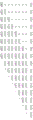•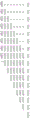•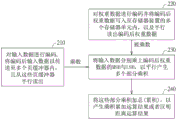•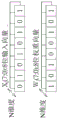•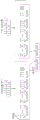•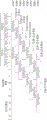•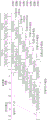•••••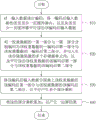## Classifications

• GPHYSICS
• G06COMPUTING; CALCULATING OR COUNTING
• G06FELECTRIC DIGITAL DATA PROCESSING
• G06F7/00Methods or arrangements for processing data by operating upon the order or content of the data handled
• G06F7/38Methods or arrangements for performing computations using exclusively denominational number representation, e.g. using binary, ternary, decimal representation
• G06F7/48Methods or arrangements for performing computations using exclusively denominational number representation, e.g. using binary, ternary, decimal representation using non-contact-making devices, e.g. tube, solid state device; using unspecified devices
• G06F7/52Multiplying; Dividing
• G06F7/523Multiplying only
• G06F7/527Multiplying only in serial-parallel fashion, i.e. one operand being entered serially and the other in parallel
• G06F7/5272Multiplying only in serial-parallel fashion, i.e. one operand being entered serially and the other in parallel with row wise addition of partial products
• GPHYSICS
• G06COMPUTING; CALCULATING OR COUNTING
• G06FELECTRIC DIGITAL DATA PROCESSING
• G06F12/00Accessing, addressing or allocating within memory systems or architectures
• G06F12/08Addressing or allocation; Relocation in hierarchically structured memory systems, e.g. virtual memory systems
• G06F12/0802Addressing of a memory level in which the access to the desired data or data block requires associative addressing means, e.g. caches
• G06F12/0877Cache access modes
• G06F12/0882Page mode
• GPHYSICS
• G06COMPUTING; CALCULATING OR COUNTING
• G06FELECTRIC DIGITAL DATA PROCESSING
• G06F13/00Interconnection of, or transfer of information or other signals between, memories, input/output devices or central processing units
• G06F13/14Handling requests for interconnection or transfer
• G06F13/16Handling requests for interconnection or transfer for access to memory bus
• G06F13/1668Details of memory controller
• G06F13/1673Details of memory controller using buffers
• GPHYSICS
• G06COMPUTING; CALCULATING OR COUNTING
• G06FELECTRIC DIGITAL DATA PROCESSING
• G06F7/00Methods or arrangements for processing data by operating upon the order or content of the data handled
• G06F7/38Methods or arrangements for performing computations using exclusively denominational number representation, e.g. using binary, ternary, decimal representation
• G06F7/48Methods or arrangements for performing computations using exclusively denominational number representation, e.g. using binary, ternary, decimal representation using non-contact-making devices, e.g. tube, solid state device; using unspecified devices
• G06F7/544Methods or arrangements for performing computations using exclusively denominational number representation, e.g. using binary, ternary, decimal representation using non-contact-making devices, e.g. tube, solid state device; using unspecified devices for evaluating functions by calculation
• G06F7/5443Sum of products
• GPHYSICS
• G06COMPUTING; CALCULATING OR COUNTING
• G06FELECTRIC DIGITAL DATA PROCESSING
• G06F7/00Methods or arrangements for processing data by operating upon the order or content of the data handled
• G06F7/60Methods or arrangements for performing computations using a digital non-denominational number representation, i.e. number representation without radix; Computing devices using combinations of denominational and non-denominational quantity representations, e.g. using difunction pulse trains, STEELE computers, phase computers
• G06F7/72Methods or arrangements for performing computations using a digital non-denominational number representation, i.e. number representation without radix; Computing devices using combinations of denominational and non-denominational quantity representations, e.g. using difunction pulse trains, STEELE computers, phase computers using residue arithmetic
• G06F7/729Methods or arrangements for performing computations using a digital non-denominational number representation, i.e. number representation without radix; Computing devices using combinations of denominational and non-denominational quantity representations, e.g. using difunction pulse trains, STEELE computers, phase computers using residue arithmetic using representation by a residue number system
• YGENERAL TAGGING OF NEW TECHNOLOGICAL DEVELOPMENTS; GENERAL TAGGING OF CROSS-SECTIONAL TECHNOLOGIES SPANNING OVER SEVERAL SECTIONS OF THE IPC; TECHNICAL SUBJECTS COVERED BY FORMER USPC CROSS-REFERENCE ART COLLECTIONS [XRACs] AND DIGESTS
• Y02TECHNOLOGIES OR APPLICATIONS FOR MITIGATION OR ADAPTATION AGAINST CLIMATE CHANGE
• Y02DCLIMATE CHANGE MITIGATION TECHNOLOGIES IN INFORMATION AND COMMUNICATION TECHNOLOGIES [ICT], I.E. INFORMATION AND COMMUNICATION TECHNOLOGIES AIMING AT THE REDUCTION OF THEIR OWN ENERGY USE
• Y02D10/00Energy efficient computing, e.g. low power processors, power management or thermal management

## Abstract

The present disclosure provides memory devices and methods of operating the same. The operation method of the memory device comprises the following steps: encoding an input data, transmitting an encoded input data into at least one page buffer, and reading the encoded input data in parallel from the at least one page buffer; encoding a first portion and a second portion of a weight data into an encoded first portion of the weight data and an encoded second portion of the weight data, respectively, writing the encoded first portion of the weight data and the encoded second portion of the weight data into a plurality of memory cells of the memory device, and reading the encoded first portion of the weight data and the encoded second portion of the weight data in parallel; multiplying the encoded input data by the encoded first portion of the weight data and the encoded second portion of the weight data, respectively, to generate a plurality of partial products in parallel; and accumulating the partial products to generate an operation result.

## Description

Memory device and method of operating the same
Technical Field
The present disclosure relates to a Memory device having In-Memory-Computing (IMC) and a method of operating the same.
Background
Artificial Intelligence (AI) has become a highly effective solution in many areas. The key operation of AI is to multiply-and-accumulate (MAC) a large amount of input data (e.g., input signature (input feature maps)) with weight values.
However, with the current AI architecture, input-output bottleneck (IO bottleneck) and low-efficiency MAC operation flow are easily encountered.
To achieve high accuracy, MAC operations with multi-bit inputs and multi-bit weight values may be performed. However, the input-output bottleneck becomes more severe and the efficiency will be lower.
In-Memory-Computing (IMC) may be used to speed up MAC operations, as IMC may reduce the complex arithmetic logic units (Arithmetic logic unit, ALU) required under a central processing architecture and provide high parallelism (parallelism) of In-Memory MAC operations.
In IMC, unsigned integer multiplication and signed integer multiplication are described below.
For example, it is desired to multiply two unsigned numbers (both 8 bits): a [7:0] and b [7:0]. Then 8 single bit multiplications may be performed to generate 8 partial products (partial products) p0[7:0] p7[7:0], each of the 8 partial products being associated with a respective bit of the multiplicand a, the 8 partial products being represented as follows:
p0[7:0]＝a×b[7:0]＝{8{a}}&b[7:0]
p1[7:0]＝a×b[7:0]＝{8{a}}&b[7:0]
p2[7:0]＝a×b[7:0]＝{8{a}}&b[7:0]
p3[7:0]＝a×b[7:0]＝{8{a}}&b[7:0]
p4[7:0]＝a×b[7:0]＝{8{a}}&b[7:0]
p5[7:0]＝a×b[7:0]＝{8{a}}&b[7:0]
p6[7:0]＝a×b[7:0]＝{8{a}}&b[7:0]
p7[7:0]＝a×b[7:0]＝{8{a}}&b[7:0]
where {8{ a  } represents repeating a  8 times, the remainder being so forth.
To obtain the product, the 8 partial products p0[7:0] to p7[7:0] are added, as shown in FIG. 1A. FIG. 1A is a multiplication of two unsigned numbers (8 bits each).
Where p0=p0  +0+0+0+0+0+0+0, and p1=p0  +p1 +0+0+0+0+0+0, the remainder being so forth.
The product P [15:0] is obtained by taking P0 to P15. The product P [15:0] represents a 16-bit unsigned product obtained by multiplying two unsigned numbers (both 8 bits).
If b is a signed number, then the partial product needs to be sign-extended to the product width before summing. If a is also a signed number, the partial product P7 is subtracted from the final sum instead of added.
FIG. 1B shows the multiplication of two symbol numbers (8 bits). In FIG. 1B, the symbols "-" represent complements, e.g., -p 1 represents the complement of p1[7 ].
In performing IMC, it would be beneficial to IMC performance if the "operating speed" could be increased and the capacity requirements (capacity requirement) reduced.
Disclosure of Invention
According to an example of the present disclosure, there is provided a memory device including: the memory device comprises a plurality of memory dies, a plurality of page buffers and an accumulation circuit, wherein each memory die comprises a plurality of memory planes, and each memory plane comprises a plurality of memory units. Wherein, encode an input data, transmit an input data after encoding to at least one page buffer, and read out the input data after encoding from the at least one page buffer in parallel; encoding a first portion and a second portion of a weight data into an encoded first portion of the weight data and an encoded second portion of the weight data, respectively, writing the encoded first portion and the encoded second portion of the weight data into the memory cells of the memory device, and reading the encoded first portion of the weight data and the encoded second portion of the weight data in parallel; multiplying the encoded input data by the encoded first portion of the weight data and the encoded second portion of the weight data, respectively, to generate a plurality of partial products in parallel; and accumulating the partial products to generate an operation result.
According to another example of the present disclosure, there is provided a method of operating a memory device, comprising: encoding an input data, transmitting an encoded input data into at least one page buffer, and reading the encoded input data in parallel from the at least one page buffer; encoding a first portion and a second portion of a weight data into an encoded first portion of the weight data and an encoded second portion of the weight data, respectively, writing the encoded first portion of the weight data and the encoded second portion of the weight data into a plurality of memory cells of the memory device, and reading the encoded first portion of the weight data and the encoded second portion of the weight data in parallel; multiplying the encoded input data by the encoded first portion of the weight data and the encoded second portion of the weight data, respectively, to generate a plurality of partial products in parallel; and accumulating the partial products to generate an operation result.
For a better understanding of the above and other aspects of the disclosure, reference will now be made in detail to the following examples, which are illustrated in the accompanying drawings:
drawings
Fig. 1A is a multiplication of two unsigned numbers.
Fig. 1B is a multiplication of two symbol numbers.
FIG. 2 is a flow chart of a method of operating a memory device according to an embodiment of the present disclosure.
Fig. 3A and 3B are diagrams illustrating error-tolerant data encoding in an embodiment of the disclosure.
Fig. 4A is an 8-bit unsigned number multiplication in an embodiment of the present disclosure.
Fig. 4B is an 8-bit signed number multiplication operation in an embodiment of the present disclosure.
Fig. 5A is an operational diagram of an unsigned number multiplication operation according to an embodiment of the present disclosure.
Fig. 5B is an operational diagram of a signed number multiplication operation according to an embodiment of the disclosure.
FIG. 6 is a functional block diagram of a memory device according to an embodiment of the present disclosure.
Fig. 7 is a timing diagram comparing a MAC operation flow of an embodiment of the present disclosure with that of the prior art.
FIG. 8 is a method of operating a memory device according to an embodiment of the present disclosure.
Description of the reference numerals
210-240: step (a)
600: memory device 615: memory die
620: memory plane 625: page buffer
630: the accumulating circuit 631: induction circuit
633-641: latch units 643, 645: logic gate
651: a partial product accumulation unit 653: single dimension product generating unit
655: first multidimensional accumulating unit
657: second multidimensional accumulating unit
659: weight accumulation control unit
810-840: step (a)
Detailed Description
Technical terms of the present specification are described or defined with reference to terms commonly used in the art, and the explanation of the terms in this section is based on the description or definition of the present specification. Various embodiments of the present disclosure each have one or more technical features. Those skilled in the art may selectively implement some or all of the features of any of the embodiments, or may selectively combine some or all of the features of the embodiments, as the implementation may suggest themselves.
FIG. 2 is a flow chart of a method of operating a memory device according to an embodiment of the present disclosure. In step 210, the input data is encoded and the encoded input data (which is a vector) is transferred into a plurality of page buffers and read out from the page buffers in parallel. Details of how the input data is encoded will be described below.
In step 220, the weight data is encoded and the encoded weight data (which is a vector) is written into a plurality of memory cells of the memory device, and the encoded weight data is read out in parallel. Details of how the weight data is encoded will be described below. Wherein, when encoding, the most significant bit (most significant bit, MSB) and the least significant bit (least significant bit, LSB) of the weight data are encoded separately.
In step 230, the input data is multiplied by MSBs and LSBs of the encoded weight data, respectively, to generate a plurality of partial products (partial products) in parallel.
In step 240, these partial products are summed (accumulated) to produce a multiply-and-accumulate (MAC) result or a Hamming distance (Hamming distance) result.
An embodiment of the present disclosure provides a memory device that can implement multi-bit MAC operations with error-bit-tolerant (error-tolerant) data encoding to tolerate error bits and reduce area requirements. Error tolerant data encoding uses input data replication (duplication) and weighting data flattening (flattening) techniques. In addition, the sensing technique of the disclosed embodiments includes a standard single-level cell (single level cell, SLC) read AND logic AND (AND) function, with bit multiplication to produce a partial product. In other possible embodiments of the present disclosure, if the page buffer does not remove the input data present in the latch Cell during sensing, the SLC read may be replaced by a select bit read or by a Multi-Level Cell (MLC), tri-Level Cell (Triple Level Cell, TLC), quad-Level Cell (QLC) read operation. In addition, the multi-bit MAC operation of one embodiment of the present disclosure uses a high bandwidth weight accumulator (high bandwidth weighted accumulator) that performs weighted accumulation (weighted accumulation) by reusing fail-bit-count (FBC) circuitry to generate the output result.
Another embodiment of the present disclosure provides a memory device capable of performing hamming distance operations with error bit tolerant data encoding to tolerate error bits. Error-tolerant data encoding uses input data replication and weight data flattening techniques. In addition, the sensing technique of embodiments of the present disclosure includes a standard single-layer cell (single level cell, SLC) read and logical exclusive or (EXOR) function, with bit multiplication to produce a partial product. In other possible embodiments of the present disclosure, the SLC read may be replaced by a select bit read or by a MLC, TLC, QLC read operation if the page buffer does not remove the input data present within the latch cell during sensing. While the logical exclusive or (EXOR) function may be replaced by a logical exclusive nor (XNOR) and a logical exclusive. In addition, the multi-bit Hamming distance operation of one embodiment of the present disclosure uses a high bandwidth unweighted accumulator (high bandwidth unweighted accumulator) that performs unweighted accumulation (unweighted accumulation) by reusing fail-bit-count (FBC) circuitry.
Fig. 3A and 3B are diagrams illustrating error-tolerant data encoding in an embodiment of the disclosure. For example, but not limited to, the input data and the weight data are floating point 32 (floating point 32) data. In fig. 3A, the input data and the weight data are quantized into 8-bit binary integers, wherein the input data and the weight data are both 8-bit vectors and are N-dimensional (N is a positive integer). The input data and the weight data can be expressed as X i (7:0) and W i (7:0)。
In fig. 3B, each 8-bit weight vector of these N dimensions is separated into an MSB vector and an LSB vector. The MSB vector of the 8-bit weight vector includes 4-bit W i (7:4) and the LSB vector comprises 4 bits W i (3:0)。
Next, each bit of the MSB vector and the LSB vector of the 8-bit weight vector is represented in Unary coding (i.e., value format). For example, bit W of MSB vector of 8-bit weight vector i =0 (7) can be expressed as 8 bits (copied 8 times), bit W of MSB vector of 8-bit weight vector i =0 (6) can be expressed as 4 bits (4 copies), bit W of MSB vector of 8-bit weight vector i =0 (5) can be expressed as 2 bits (2 copies), bit W of MSB vector of 8-bit weight vector i =0 (4) can be expressed as 1 bit (copy 1 time), and spare bit (0) is added to bit W of MSB vector of 8-bit weight vector i After=0 (4). In this way, the 4-bit MSB vector of the 8-bit weight vector may be encoded into a 16-bit Unary coding (Unarry coding) format.
Likewise, a 4-bit LSB vector of an 8-bit weight vector may be encoded into a 16-bit Unary coding (Unary coding) format.
In an embodiment of the present disclosure, the tolerance of the error bits may be improved by the last encoding method.
Fig. 4A is an 8-bit unsigned integer multiplication in an embodiment of the present disclosure, and fig. 4B is an 8-bit signed integer multiplication in an embodiment of the present disclosure.
As shown in FIG. 4A, when 8-bit unsigned number multiplication is performed, X of the input data is input at the 0 th cycle i (7) MSB vector W of (input data has been encoded into unary encoding format) multiplied by weight data i (7:4) (the MSB vector of the weight data has been encoded into a unary encoding format) to obtain a first MSB partial product. Similarly, X of the input data i (7) LSB vector W multiplied by weight data i (3:0) (the LSB vector of the weight data has been encoded into a unary encoding format) to obtain a first LSB partial product. The first MSB partial product is shifted by 4 bits and added to the first LSB partial product to obtain the first partial product.
At 1 st cycle, X of the input data i (6) Multiplying MSB vector W of weight data i (7:4) (the MSB vector of the weight data has been encoded into a unary coding format) to obtain a second MSB partial product. Similarly, X of the input data i (6) LSB vector W multiplied by weight data i (3:0) (the LSB vector of the weight data has been encoded into a unary encoding format) to obtain a second LSB partial product. The second MSB partial product is shifted by 4 bits and added to the second LSB partial product to obtain the second partial product. In addition, the first partial product is shifted by 1 bit and added to the second partial product to obtain the updated second partial product. The operations of the remaining periods (period 2 to period 7) can be analogized and are not repeated here.
That is, an 8-bit unsigned number multiplication operation can be completed by 8 cycles.
As shown in FIG. 4B, when 8-bit signed number multiplication is performed, X of the input data is input at the 0 th cycle i (7) Multiplying MSB vector W of weight data i (7) (the MSB vector of the weight data has been encoded into a unary encoding format), and X of the input data i (7) Multiplying MSB vector W of weight data i (6: 4) (the MSB vectors of the weight data have been encoded into a unitary encoding format) and inverted, and the two are added to obtain a first MSB partial product. X to input data i (7) LSB vector W multiplied by weight data i (3:0) (the LSB vector of the weight data has been encoded into a unary encoding format) and inverted to obtain a first LSB partial product. The first MSB partial product is shifted by 4 bits and added to the first LSB partial product to obtain the first partial product.
At 1 st cycle, X of the input data i (6) Multiplying MSB vector W of weight data i (7) (the MSB vector of the weight data has been encoded into the unary encoding format) and then inverted, and X of the input data is calculated i (6) Multiplying MSB vector W of weight data i (6: 4) (the MSB vectors of the weight data have been encoded into a unitary encoding format) and added to obtain a second MSB partial product. Similarly, X of the input data i (6) LSB vector W multiplied by weight data i (3:0) (weightThe LSB vector of the data has been encoded into a unary encoding format) to obtain a second LSB partial product. The second MSB partial product is shifted by 4 bits and added to the second LSB partial product to obtain the second partial product. In addition, the first partial product is shifted by 1 bit and added to the second partial product to obtain the updated second partial product. The operations of the remaining periods (period 2 to period 7) can be analogized and are not repeated here.
That is, an 8-bit signed number multiplication operation can be completed by 8 cycles.
The above-described manner requires 8 cycles to complete an 8-bit unsigned number multiplication and an 8-bit signed number multiplication.
Fig. 5A is an operational diagram of an unsigned number multiplication operation according to an embodiment of the present disclosure. Fig. 5B is an operational diagram of a signed number multiplication operation according to an embodiment of the disclosure. Fig. 5A and 5B illustrate an example in which the input data and the weight data are 8 bits, but it should be understood that the disclosure is not limited thereto.
In fig. 5A and 5B, the input data is also encoded, and the MSB vector and LSB vector of the weight data are encoded into a unitary encoding format.
In fig. 5A and 5B, the input data is input to the page buffer, and the weight data is written into the plurality of memory cells.
In fig. 5A, input data is read out from the page buffer in parallel, and weight data is read out from these memory cells in parallel, and parallel multiplication is performed to obtain partial products.
Specifically, bit X of the input data i (7) Multiplying MSB vector W of weight data i (7:4) to obtain a first MSB partial product. Bit X of input data i (6) Multiplying MSB vector W of weight data i (7:4) to obtain a second MSB partial product. The rest can be analogized to bit X of the input data i (0) Multiplying MSB vector W of weight data i (7:4) to obtain an eighth MSB partial product. For example, in FIG. 5A, bit X of the input data i (7) Is copied 15 times and spare bits are added to become a 16-bit multiplier "0000000000000000". This 16-bit multiplier"0000000000000000" multiplied by MSB vector W of weight data i (7:4) "1111111100001100" to obtain the first MSB partial product "0000000000000000". The rest may be so on. All MSB partial products can be combined into an input stream (input stream) M.
Similarly, X of the input data i (7) LSB vector W multiplied by weight data i (3:0) to obtain a first LSB partial product. X to input data i (6) LSB vector W multiplied by weight data i (3:0) to obtain a second LSB partial product. The rest can be analogized to bit X of the input data i (0) LSB vector W multiplied by weight data i (3:0) to obtain an eighth LSB partial product. All LSB partial products may be combined into the input stream L.
And combining the first to eighth MSB partial products with the first to eighth LSB partial products, and counting the number of bit 1 of the combined value to obtain the MAC operation result of the unsigned number multiplication operation.
In fig. 5B, input data is read out from the page buffer in parallel, and weight data is read out from these memory cells in parallel, and parallel multiplication is performed to obtain partial products.
Specifically, bit X of the input data i (7) Multiplying MSB vector W of weight data i (7:4) to obtain a first MSB partial product. Bit X of input data i (6) Multiplying MSB vector W of weight data i (7:4) to obtain a second MSB partial product. The rest can be analogized to bit X of the input data i (0) Multiplying MSB vector W of weight data i (7:4) to obtain an eighth MSB partial product.
Similarly, X of the input data i (7) LSB vector W multiplied by weight data i (3:0) to obtain a first LSB partial product. X to input data i (6) LSB vector W multiplied by weight data i (3:0) to obtain a second LSB partial product. The rest can be analogized to bit X of the input data i (0) LSB vector W multiplied by weight data i (3:0) to obtain an eighth LSB partial product.
And combining the first to eighth MSB partial products with the first to eighth LSB partial products, and counting the number of bit 1 of the combined value to obtain the MAC operation result of the signed multiplication operation.
FIG. 6 is a functional block diagram of a memory device according to an embodiment of the present disclosure. The memory device 600 includes a plurality of memory dies (die) 615. In fig. 6, the memory device 600 is illustrated as including 4 memory dies 615, but it is understood that the present disclosure is not limited thereto.
Memory die 615 includes a plurality of Memory Planes (MPs) 620, a plurality of page buffers 625, and an accumulation circuit 630. In fig. 6, the memory die 615 is illustrated as including 4 memory planes 620 and 4 page buffers 625, but it is understood that the disclosure is not limited thereto. Memory plane 620 includes a plurality of memory cells (not shown). Weight data is stored in these memory cells.
Within each memory die 615, the accumulation circuit 630 is shared by the memory planes 620, so that the accumulation circuit 630 sequentially performs the accumulation operations for the memory planes 620. In addition, each memory die 615 may independently perform the multi-bit MAC operations and multi-bit hamming distance operations described above for embodiments of the present disclosure.
Input data may be input into these page buffers 625 through a plurality of word lines.
The page buffer 625 includes a sensing circuit 631, a plurality of latch units 633-641, and a plurality of logic gates 643 and 645.
The sensing circuit 631 is coupled to the bit line BL to sense a current on the bit line BL.
The latch units 633-641 are, for example and without limitation, data Latch (DL) 633, latch (L1) 635, latch (L2) 637, latch (L3) 639, and common data latch (common data latch, CDL) 641, respectively. The latch units 633-641 are, for example, but not limited to, single layer latches.
The data latch 633 is used to latch the weight data and outputs the weight data to the logic gates 643 and 645.
Latch (L1) 635 and latch (L3) 639 are used for decoding.
The latch (L2) 637 latches the input data and outputs the input data to the logic gates 643 and 645.
The common data latch 641 is used for latching the data transmitted from the logic gate 643 or 645.
Logic gates 643 and 645 are, for example and without limitation, logic and gates, and logic XOR gates, respectively. The logic gate 643 performs a logical and operation on the input data and the weight data, and writes the result of the logical operation to the common data latch 641. The logic gate 645 performs a logic XOR operation on the input data and the weight data, and writes the result of the logic operation to the common data latch 641. Logic gates 643 AND 645 are controlled by enable signals and_en AND xor_en, respectively. For example, when performing a multi-bit MAC operation, logic gate 643 is enabled by enable signal and_en; and, when performing a multi-bit hamming distance operation, logic gate 645 is enabled by an enable signal xor_en.
With reference to FIG. 5A or FIG. 5B, bit X of the input data is illustrated i (7) Is input to a latch (L2) 637, and is encoded into an MSB vector W of weight data in a unitary encoding format i (7:4) 1 bit is input to the data latch 633. The input data of the latch (L2) 637 and the weight data of the data latch 633 are logically operated by the logic gate 643 or 645, and then the common data latch 641 is used for latching the data transmitted from the logic gate 643 or 645. The common data latch 641 may also be referred to as a data output path for the bit line.
The accumulation circuit 630 includes: a partial product accumulation unit 651, a single-dimensional product generation unit 653, a first multi-dimensional accumulation unit 655, a second multi-dimensional accumulation unit 657, and a weight accumulation control unit 659.
The partial product accumulating unit 651 is coupled to the page buffer 625 for receiving the logic operation results from the common data latches 641 of the page buffer 625 to generate partial products.
For example, in fig. 5A or 5B, the partial product accumulating unit 651 generates the first to eighth MSB partial products and the first to eighth LSB partial products.
The single-dimensional product generating unit 653 is coupled to the partial product accumulating unit 651, and accumulates the partial products generated by the partial product accumulating unit 651 to generate a single-dimensional product.
For example, referring to fig. 5A or 5B, the single-dimensional product generating unit 653 sums the first to eighth MSB partial products and the first to eighth LSB partial products generated by the partial product accumulating unit 651 to generate a single-dimensional product.
For example, after the <0> dimensional product is generated in the 0 th period, the <1> dimensional product may be generated in the 1 st period, and so on.
The first multi-dimensional accumulating unit 655 is coupled to the single-dimensional product generating unit 653, and accumulates the plurality of single-dimensional products generated by the single-dimensional product generating unit 653 to obtain a multi-dimensional product accumulating result.
For example, but not limited to, the first multi-dimensional accumulating unit 655 accumulates the <0> to <7> dimensional products generated by the single-dimensional product generating unit 653 to obtain an 8-dimensional <0:7> product accumulation result. Next, the first multi-dimensional accumulating unit 655 accumulates the <8> to <15> dimensional products generated by the single-dimensional product generating unit 653 to obtain another 8-dimensional <8:15> dimensional product accumulation result.
The second multi-dimensional accumulating unit 657 is coupled to the first multi-dimensional accumulating unit 655, and accumulates the multi-dimensional product accumulating results generated by the first multi-dimensional accumulating unit 655 to obtain an output accumulated value. For example, but not limited to, the second multi-dimensional accumulating unit 657 accumulates the 64 8-dimensional multiply-accumulate results generated by the first multi-dimensional accumulating unit 655 to obtain 512-dimensional output accumulated values.
The weight accumulation control unit 659 is coupled to the partial product accumulation unit 651, the single-dimensional product generation unit 653, and the first multi-dimensional accumulation unit 655. The weight accumulation control unit 659 is enabled or disabled according to whether a multi-bit MAC operation or a multi-bit hamming distance operation is performed. For example, but not limited to, when performing a multi-bit MAC operation, the weight accumulation control unit 659 is enabled; and, when the multi-bit hamming distance operation is performed, the weight accumulation control unit 659 is disabled. When the weight accumulation control unit 659 is enabled, the weight accumulation control unit 659 outputs a control signal to the partial product accumulation unit 651, the single-dimensional product generation unit 653, and the first multi-dimensional accumulation unit 655 according to the weight accumulation enable signal wacc_en.
The single page buffer 620 in fig. 6 is coupled to a plurality of bit lines BL. For example, but not limited to, each page buffer 620 is coupled to 131072 bit lines BL, and the data result on 128 bit lines BL is selected for accumulation in the accumulation circuit 630 in each cycle. In this way, 1024 cycles are required to complete the data on 131072 bit lines BL.
Also in the above description, the partial product accumulation unit 651 receives 128 bits at a time, the first multi-dimensional accumulation unit 655 generates an 8-dimensional product accumulation result, and the second multi-dimensional accumulation unit 657 generates an output accumulation value of 512 dimensions. The present disclosure is not so limited. In another possible embodiment, the partial product accumulation unit 651 receives 64 bits at a time (2 bits are 1 group), the first multi-dimensional accumulation unit 655 generates a 16-dimensional product accumulation result, and the second multi-dimensional accumulation unit 657 generates a 512-dimensional output accumulation value.
Fig. 7 is a timing diagram comparing a MAC operation flow of an embodiment of the present disclosure with that of the prior art. Referring to fig. 7, input data is received during an input broadcast (input broadcasting) time. Then, the input data and the weight data are subjected to bit multiplication and bit accumulation in the manner described above to generate a MAC operation result.
In the prior art, a long operation time is required. In contrast, in the presently disclosed embodiments, the partial product of (1) the input vector and the MSB vector of the weight data, and (2) the partial product of the input vector and the LSB vector of the weight data are generated by parallel multiplication. Thus, the unsigned number multiplication and/or signed number multiplication can be completed within 1 period. Therefore, the embodiments of the present disclosure operate faster than the prior art.
FIG. 8 is a method of operating a memory device according to an embodiment of the present disclosure, comprising: encoding an input data, transferring an encoded input data into at least one page buffer, and reading the encoded input data in parallel from the at least one page buffer (810); encoding a first portion and a second portion of a weight data into an encoded first portion of the weight data and an encoded second portion of the weight data, respectively, and writing into a plurality of memory cells of the memory device, and reading out the encoded first portion of the weight data and the encoded second portion of the weight data in parallel (820); multiplying the encoded input data by the encoded first portion of the weight data and the encoded second portion of the weight data, respectively, to generate a plurality of partial products in parallel (830); and accumulating the partial products to generate an operation result (840).
As described above, in the embodiments of the present disclosure, error bits can be reduced, accuracy can be improved, and memory capacity requirements can be reduced by bit tolerant encoding.
In addition, the multi-bit MAC operation of one embodiment of the present disclosure uses a high bandwidth weight accumulator to generate the output result, and the high bandwidth weight accumulator can implement weighted accumulation by reusing the fault bit counting circuit, so that the accumulation speed can be improved.
In one embodiment of the present disclosure, the multi-bit Hamming distance operation uses a high bandwidth unweighted accumulator to generate the output result, and the high bandwidth unweighted accumulator can implement unweighted accumulation by reusing the fault bit counting circuit, so that the accumulation speed can be improved.
The above embodiments of the present disclosure may be applied to NAND type flash memory, or memory devices sensitive to erroneous bits, such as, but not limited to, NOR type flash memory, phase Change (PCM) type flash memory, magnetic random access memory (magnetic RAM) or resistive RAM.
In the above embodiment, the accumulation circuit 630 may receive 128 partial products from the page buffer 625, but in other embodiments of the disclosure, the accumulation circuit 630 may receive 2, 4, 8, 16, … partial products (to the power of 2) from the page buffer 625, which is also within the spirit of the disclosure.
In the above embodiments, the accumulating circuit 630 may support the addition function, but in other embodiments of the present disclosure, the accumulating circuit 630 may support the subtraction function, which is also within the spirit of the present disclosure.
Although the embodiments described above take the MAC operations of INT8 or UNIT8 as examples, in other embodiments of the disclosure, the MAC operations of INT2, UNIT2, INT4, UNIT4 may also be supported, which is within the scope of the present disclosure.
Although in the above-described embodiment, the weight data is divided into the MSB vector and the LSB vector (2 vectors), the present disclosure is not limited thereto. In other possible embodiments of the present disclosure, the weight data may be divided into more vectors, which are also within the spirit of the present disclosure.
The above embodiments of the disclosure may be applied to AI model designs requiring MAC operation, such as, but not limited to, AI techniques including full-connection layer (full-connection layer), convolution layer (convolution layer), multi-layer perceptron (multiple layer Perceptron), support vector machine (support vector machine), etc.
The present disclosure is applicable not only to computing use (computing use), but also to similarity search (similarity search), analysis use (analysis use), cluster analysis (clustering analysis), and the like.
In summary, although the disclosure has been disclosed in terms of embodiments, it is not intended to limit the disclosure. Those skilled in the art to which the present disclosure pertains will appreciate that numerous modifications and variations can be made without departing from the spirit and scope of the disclosure. Accordingly, the scope of the present disclosure is defined by the scope of the appended claims.

## Claims (10)

1. A memory device, comprising:
a plurality of memory dies, each of the memory dies including a plurality of memory planes, a plurality of page buffers, and an accumulation circuit, each of the memory planes including a plurality of memory cells,
wherein, encode an input data, transmit an input data after encoding to at least one page buffer, and read out the input data after encoding from the at least one page buffer in parallel;
encoding a first portion and a second portion of a weight data into an encoded first portion of the weight data and an encoded second portion of the weight data, respectively, writing the encoded first portion and the encoded second portion of the weight data into the memory cells of the memory device, and reading the encoded first portion of the weight data and the encoded second portion of the weight data in parallel;
multiplying the encoded input data by the encoded first portion of the weight data and the encoded second portion of the weight data, respectively, to generate a plurality of partial products in parallel; and
the partial products are accumulated to produce an operation result.
2. The memory device of claim 1, wherein,
the first portion of the weight data is the most significant bits and the second portion of the weight data is the least significant bits.
3. The memory device of claim 1, wherein,
during encoding, respectively quantizing the input data and the weight data into binary integer vectors;
copying each bit of the input data multiple times and adding a spare bit;
splitting the weight data into the first portion and the second portion; and
and representing each bit of the first part and the second part of the weight data by unitary coding to obtain the coded first part of the weight data and the coded second part of the weight data.
4. The memory device of claim 1, wherein,
the operation result comprises a product accumulation result operation result or a hamming distance operation result; the method comprises the steps of,
accumulating the partial products belonging to the same dimension to obtain a single-dimension product;
accumulating the products of the multiple single dimensions to obtain a multi-dimensional product accumulation result;
and accumulating a plurality of multi-dimensional product accumulation results to generate the operation result.
5. The memory device of claim 4, wherein,
when the multiply-accumulate operation is performed, performing a logical AND operation on each bit of the encoded input data and each bit of the encoded first portion of the weight data; and
when Hamming distance operation is performed, logical exclusive OR operation is performed on each bit of the encoded input data and each bit of the encoded first portion of the weight data.
6. A method of operation of a memory device, comprising:
encoding an input data, transmitting an encoded input data into at least one page buffer, and reading the encoded input data in parallel from the at least one page buffer;
encoding a first portion and a second portion of a weight data into an encoded first portion of the weight data and an encoded second portion of the weight data, respectively, writing the encoded first portion of the weight data and the encoded second portion of the weight data into a plurality of memory cells of the memory device, and reading the encoded first portion of the weight data and the encoded second portion of the weight data in parallel;
multiplying the encoded input data by the encoded first portion of the weight data and the encoded second portion of the weight data, respectively, to generate a plurality of partial products in parallel; and
the partial products are accumulated to produce an operation result.
7. The method of operation of a memory device of claim 6, wherein,
the first portion of the weight data is the most significant bits and the second portion of the weight data is the least significant bits.
8. The method of operation of a memory device of claim 6, wherein,
during encoding, respectively quantizing the input data and the weight data into binary integer vectors;
copying each bit of the input data multiple times and adding a spare bit;
splitting the weight data into the first portion and the second portion; and
and representing each bit of the first part and the second part of the weight data by unitary coding to obtain the coded first part of the weight data and the coded second part of the weight data.
9. The method of operation of a memory device of claim 6, wherein,
the operation result comprises a product accumulation result operation result or a hamming distance operation result; and
accumulating the partial products belonging to the same dimension to obtain a single-dimension product;
accumulating the products of the multiple single dimensions to obtain a multi-dimensional product accumulation result;
and accumulating a plurality of multi-dimensional product accumulation results to generate the operation result.
10. The method of operation of a memory device of claim 9, wherein,
when the multiply-accumulate operation is performed, performing a logical AND operation on each bit of the encoded input data and each bit of the encoded first portion of the weight data; and
when Hamming distance operation is performed, logical exclusive OR operation is performed on each bit of the encoded input data and each bit of the encoded first portion of the weight data.
CN202210322542.5A 2021-11-22 2022-03-29 Memory device and method of operating the same Pending CN116153367A (en)

## Applications Claiming Priority (4)

Application Number Priority Date Filing Date Title
US202163281734P 2021-11-22 2021-11-22
US63/281,734 2021-11-22
US17/701,725 US20230161556A1 (en) 2021-11-22 2022-03-23 Memory device and operation method thereof
US17/701,725 2022-03-23

## Publications (1)

Publication Number Publication Date
CN116153367A true CN116153367A (en) 2023-05-23

# Family

## Family Applications (1)

Application Number Title Priority Date Filing Date
CN202210322542.5A Pending CN116153367A (en) 2021-11-22 2022-03-29 Memory device and method of operating the same

## Country Status (2)

US (1) US20230161556A1 (en)
CN (1) CN116153367A (en)

## Also Published As

Publication number Publication date
US20230161556A1 (en) 2023-05-25

## Similar Documents

Publication Publication Date Title
US20220399904A1 (en) Recurrent neural networks and systems for decoding encoded data
CN105049061A (en) Advanced calculation-based high-dimensional polarization code decoder and polarization code decoding method
US11537861B2 (en) Methods of performing processing-in-memory operations, and related devices and systems
Li et al. A stochastic reconfigurable architecture for fault-tolerant computation with sequential logic
Azamat et al. Quarry: Quantization-based ADC reduction for ReRAM-based deep neural network accelerators
Sadi et al. Accelerating deep convolutional neural network base on stochastic computing
Tsai et al. RePIM: Joint exploitation of activation and weight repetitions for in-ReRAM DNN acceleration
Ahn et al. Deeper weight pruning without accuracy loss in deep neural networks: Signed-digit representation-based approach
GB1579100A (en) Digital arithmetic method and means
Boo et al. A VLSI architecture for arithmetic coding of multilevel images
TWI796977B (en) Memory device and operation method thereof
CN116153367A (en) Memory device and method of operating the same
Zhou et al. Approximate comparator: Design and analysis
Hao et al. Stochastic-HD: leveraging stochastic computing on hyper-dimensional computing
CN113902109A (en) Compression method and device for regular bit serial computation of neural network
CN114153421A (en) Memory device and operation method thereof
CN115398392A (en) Arithmetic logic unit
Luo et al. A single clock cycle approximate adder with hybrid prediction and error compensation methods
Tang et al. A delta sigma modulator-based stochastic divider
Imani Machine learning in iot systems: From deep learning to hyperdimensional computing
Muscedere Difficult operations in the multi-dimensional logarithmic number system.
Srikanthan et al. Area-time efficient sign detection technique for binary signed-digit number system
Cardarilli et al. Approximated Canonical Signed Digit for Error Resilient Intelligent Computation
Wang et al. Design and evaluation of approximate adders
CN112949830B (en) Intelligent inference network system and addition unit and pooling unit circuitry

## Legal Events

Date Code Title Description
PB01 Publication
PB01 Publication
SE01 Entry into force of request for substantive examination
SE01 Entry into force of request for substantive examination